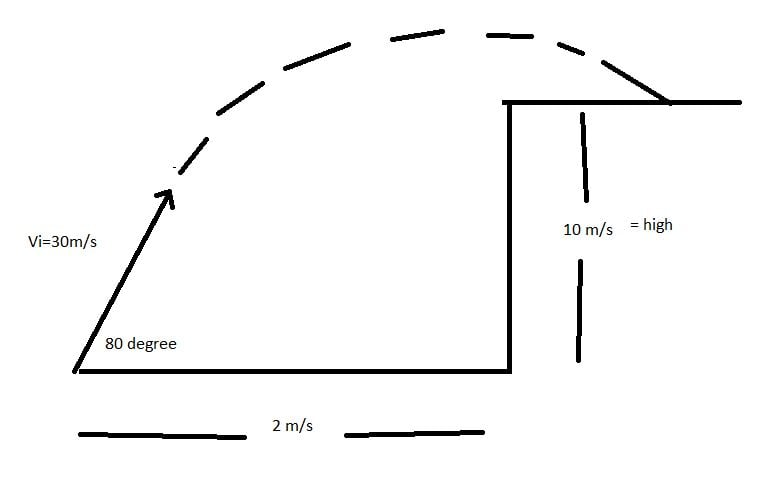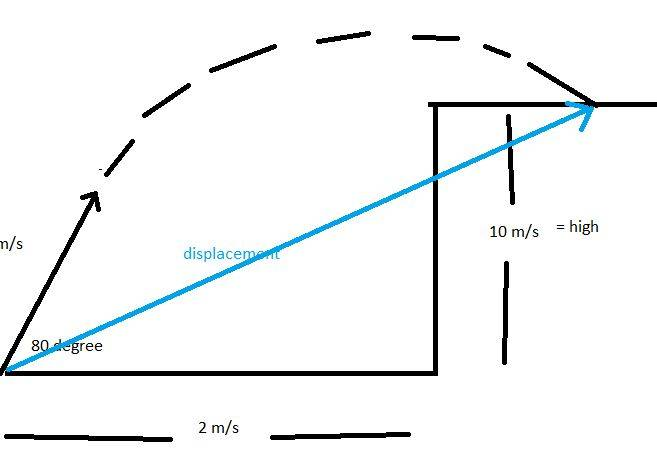# Find the rate of change of velocity

Homework Statement:
find rate of change of velocity
Relevant Equations:
Vy=vi*sin(angle)
Vx=vi*cos(angle)
Ymax=vi*t-(g*t^2)/2
Xmax=vi*t
Hello everyone,

I have a problem where I have to find the final displacement and final velocity which I have found however, I want to post few variations for that same problem which I am curious. It is more for my own knowledge > I would appreciate any helpA projectile has been launched with Initial velocity 30 m/s, 80 degree elevation, there is 2 m in the x coordinate and 10 m high

In order to find final velocity I got delta t (second grade equation)

Code:
10=29.54*t-4.9*t^2

so, t= 5.67 seconds

then, total displacement is
Code:
Sx=30*cos80 * 5.67

which is equal to 29.53
then, I used Phythagoras and I got the relationship between 29.53 and 10 m high to find the displacement
which is 31.18 myou can see the blue line

Then I can find the Vf using this idea

variation of V is equal vf-vi which is equal to g* t

then, my VF will be -26.03 m/s

Here is my question or some questions,

1. I assume is negative because the object is falling down, isn't it?
2. Then, the Vi (initial velocity) is positive because is in opposed direction, isn't it?
3. So, I think the velocity will be zero in the vertex of the parabola, isn't it
4. I think is I derive the equation for displacement , I will get the equation for velocity but what is the exact equation for displacement that represent this exercise ?
5. How can I find the vertex using the derive? Can I take displacement, then put equal to 0 and find the solution? I would need a help for this question

haruspex
Homework Helper
Gold Member
2020 Award
1. I assume is negative because the object is falling down, isn't it?
2. Then, the Vi (initial velocity) is positive because is in opposed direction, isn't it?
3. So, I think the velocity will be zero in the vertex of the parabola, isn't it
If you mean the vertical component of velocity, and you are taking up as positive, yes.
4. I think is I derive the equation for displacement , I will get the equation for velocity
I'm not sure exactly what you mean.
In the diagram you have marked the start to end displacement with a blue line. That is a constant, not a variable function of time, so deriving that will produce zero.
If you consider the displacement (which is a vector) as a function of time during the flight then, yes, the derivative of that is the velocity vector as a function of time.

But perhaps you mean vertical displacement (height) as a function of time. Deriving that will give the vertical component of velocity, and that will be zero at the apex.

Mister T
Gold Member
Homework Statement:: find rate of change of velocity
Relevant Equations:: Vy=vi*sin(angle)
Vx=vi*cos(angle)
Ymax=vi*t-(g*t^2)/2
Xmax=vi*t

How can I find the vertex using the derive?
You're looking for the event that occurs when the vertical component of the velocity is zero.

If you mean the vertical component of velocity, and you are taking up as positive, yes.

I'm not sure exactly what you mean.
In the diagram you have marked the start to end displacement with a blue line. That is a constant, not a variable function of time, so deriving that will produce zero.
If you consider the displacement (which is a vector) as a function of time during the flight then, yes, the derivative of that is the velocity vector as a function of time.

But perhaps you mean vertical displacement (height) as a function of time. Deriving that will give the vertical component of velocity, and that will be zero at the apex.

Thanks for your response. The blue line is just the total displacement but this is parabolic trajectory, for sure, there are a X and Y component.

So, what it would be the equation for the parabolic trajectory ?
With this equation I think I can implement a derive, isn't it?
So, you mentioned it is not a variable function of time. I know the delta t is 5.67 seconds. So, you mean that it is not correct apply deriving because this time will be constant during the whole process of the displacement ?
or the right questions is:
what it would be the condition or function where we could implement deriving?
Could you please provide some example?

haruspex
Homework Helper
Gold Member
2020 Award
what it would be the equation for the parabolic trajectory ?
"The equation" for the trajectory would just be y as a quadratic function of x.
If you differentiate that (with respect to x) it will give you the gradient at any particular point but will not give you a velocity.
If you mean the trajectory in parametric form, with time as the parameter, that will be two equations: y as a quadratic in t and x as a linear function of t. Differentiating those with respect to time will give you velocities.

This has little to do with the total displacement shown by your blue line, nor with the total time you calculated. I struggle to understand what you are trying to do.

Neither do I understand why you would want to "derive" (differentiate) the trajectory with respect to time. The velocity equations are easily obtained directly: vx(t)=Vcos(θ), vy(t)=Vsin(θ)-gt.

Last edited:
Mister T